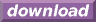#Calcute: freeware calculator

## Power(pow, ^)

A power is an operation involving two numbers: a base value and an exponent value. The operation corresponds to a multiplication of the base by its own value the number of times represented by the exponent value. Calcute implements powers with function pow which takes two parameters. This function is equivalent to using the ^ operator.

```pow(2; 4)
16

2^4
16

2*2*2*2
16
```